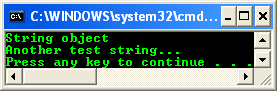3. # for each, in

The following example shows how to declare a handle to an object on the managed heap, where the type of object is a boxed value type. The sample also shows how to get the value type from the boxed object.

// The ^ handle, main project file.

#include "stdafx.h"

using namespace System;

void Test(Object^ obj)

{

// cast object to Int32...

Int32^ i = dynamic_cast<Int32^>(obj);

if(i)

Console::WriteLine("i = {0}", i);

else

Console::WriteLine("i = ?, Not a boxed int");

}

int main(array<System::String ^> ^args)

{

// string object...

String^ str = "String object test";

Test(str);

int n = 100;

// normal integer...

Test(n);

return 0;

}

Output: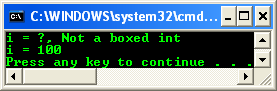The following sample shows that the common C++ idiom of using a void* pointer to point to an arbitrary object is replaced by Object^, which can hold a handle to any reference class. It also shows that all types, such as arrays and delegates, can be converted to an object handle.

// ^ handle, main project file.

#include "stdafx.h"

using namespace System;

using namespace System::Collections;

public delegate void MyDel();

ref class MyClass

{

public:

void Test() {}

};

void Test(Object ^ x)

{

Console::WriteLine("Type is {0}", x->GetType());

}

int main(array<System::String ^> ^args)

{

// handle to Object can hold any ref type

Object ^ h_MyClass = gcnew MyClass;

ArrayList ^ arr = gcnew ArrayList();

h_MyClass = dynamic_cast<MyClass ^>(arr);

Test(arr);

Int32 ^ bi = 1;

Test(bi);

MyClass ^ h_MyClass2 = gcnew MyClass;

MyDel^ DelInst = gcnew MyDel(h_MyClass2, &MyClass::Test);

Test(DelInst);

return 0;

}

Output: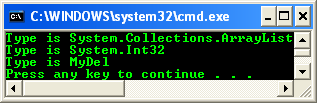The following example shows that a handle can be dereferenced and that a member can be accessed via a dereferenced handle.

// ^ handle, main project file.

#include "stdafx.h"

using namespace System;

value struct DataCollection

{

private:

int Size;

array<String^>^ x;

public:

DataCollection(int i) : Size(i)

{

x = gcnew array<String^>(Size);

for (int i = 0 ; i < Size ; i++)

x[i] = i.ToString();

}

void funct(int Item)

{

if (Item >= Size)

{

System::Console::WriteLine("Cannot access array element {0}, size is {1}", Item, Size);

return;

}

else

System::Console::WriteLine("Array value: {0}", x[Item]);

}

};

void funct(DataCollection y, int Item)

{

y.funct(Item);

}

int main(array<System::String ^> ^args)

{

DataCollection ^ a = gcnew DataCollection(10);

// dereference a handle, return handle's object

funct(*a, 7);

// access member via dereferenced handle

(*a).funct(11);

return 0;

}

Output: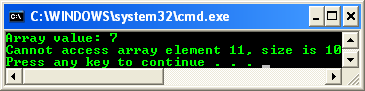The following example shows that a native reference (&) can’t bind to an int member of a managed type, as the int may be stored in the garbage collected heap, and native references don’t track object movement in the managed heap. The fix is to use a local variable, or to change & to %, making it a tracking reference.

// ^ handle, main project file.

#include "stdafx.h"

using namespace System;

ref struct myStruct

{

void Test(unsigned int &){Console::WriteLine("In Test()...");}

void Test2(unsigned int %){Console::WriteLine("In Test2()...");}

unsigned int i;

};

int main(array<System::String ^> ^args)

{

myStruct strutype;

strutype.i = 10;

// a.Test(a.i);   // C2664

strutype.Test2(strutype.i);   // OK

unsigned int j = 0;

strutype.Test(j);   // OK

return 0;

}

Output: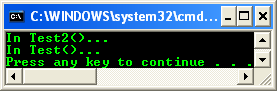gcnew

gcnew creates an instance of a managed type (reference or value type) on the garbage collected heap. The result of the evaluation of a gcnew expression is a handle (^) to the type being created. For example:

ref struct Message

{

System::String ^ sender, ^ receiver , ^ data;

};

int main(array<System::String ^> ^args)

{

Message ^ h_Message  = gcnew Message;

return 0;

}

It is possible to create an instance of a managed type, where the managed type contains a nested type other than a reference type. Consider the following program example.

// ^ handle, main project file.

#include "stdafx.h"

using namespace System;

ref class MyClass

{

public:

void Test() {}

value class Value_Nested_Class

{

public:

int i;

};

};

int main(array<System::String ^> ^args)

{

MyClass ^ h_MyClass = gcnew MyClass;

MyClass::Value_Nested_Class y;

y.i = 100;

System::Console::WriteLine("y.i = {0}", y.i);

return 0;

}

Output: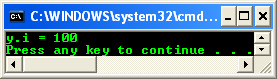for each, in

The for each statement is used to iterate through a collection of elements. The syntax is:

 Syntax for each (type identifier in expression) {statements } Parameters type The type of identifier. identifier The iteration variable that represents the collection element. When identifier is a tracking reference, you can modify the element. expression A managed array expression or collection. The compiler must be able to convert the collection element from Object to the identifier type. expression evaluates to a type that implements IEnumerable, IEnumerable, or a type that defines a GetEnumerator method. In the latter case, GetEnumerator should either return a type that implements IEnumerator or declares all the methods defined in IEnumerator. statements One or more statements to be executed. Table 16

The for each statement is used to iterate through a collection. It is possible to modify elements in a collection, but you cannot add or delete elements. The statements are executed for each element in the array or collection. After the iteration has been completed for all the elements in the collection, control is transferred to the next statement following the for each block. The following example shows how to iterate through a string with for each.

// ^ handle, main project file.

#include "stdafx.h"

using namespace System;

ref struct MyClass

{

property String ^ MyStringProperty;

};

int main(array<System::String ^> ^args)

{

String ^ MyString = gcnew String("String object");

for each (Char c in MyString)

Console::Write(c);

Console::WriteLine();

MyClass ^ x = gcnew MyClass();

x->MyStringProperty = "Another test string...";

for each(Char c in x->MyStringProperty)

Console::Write(c);

Console::WriteLine();

return 0;

}

Output: